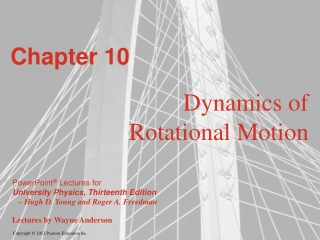DownloadDownload PresentationChapter 10

# Chapter 10

Télécharger la présentation## Chapter 10

- - - - - - - - - - - - - - - - - - - - - - - - - - - E N D - - - - - - - - - - - - - - - - - - - - - - - - - - -
##### Presentation Transcript

1. Chapter 10 Dynamics of Rotational Motion

2. Goals for Chapter 10 • To learn what is meant by torque • To see how torque affects rotational motion • To analyze the motion of a body that rotates as it moves through space • To use work and power to solve problems for rotating bodies • To study angular momentum and how it changes with time • To learn why a gyroscope precesses

3. Introduction • The north star is Polaris today, but 5000 years ago it was Thuban. What caused the change? • What causes bodies to start or stop spinning? • We’ll introduce some new concepts, such as torque and angular momentum, to deepen our understanding of rotational motion.

4. Loosen a bolt • Which of the three equal-magnitude forces in the figure is most likely to loosen the bolt?

5. Torque • The line of action of a force is the line along which the force vector lies. • The lever arm (or moment arm) for a force is the perpendicular distance from O to the line of action of the force (see figure). • The torque of a force with respect to O is the product of the force and its lever arm.

6. Torque as a vector • Torque can be expressed as a vector using the vector product. • Figure 10.4 at the right shows how to find the direction of torque using a right hand rule.

7. Applying a torque • Follow Example 10.1 using Figure 10.5.

8. Torque and angular acceleration for a rigid body • The rotational analog of Newton’s second law for a rigid body is z =Iz. • Read Problem-Solving Strategy 10.1 and apply it to Example 10.2 using Figure 10.9 below.

9. Another unwinding cable • We analyze the block and cylinder from Example 9.8 using torque. • Follow Example 10.3 using Figure 10.10.

10. Rigid body rotation about a moving axis • The motion of a rigid body is a combination of translational motion of the center of mass and rotation about the center of mass (see Figure 10.11 at the right). • The kinetic energy of a rotating and translating rigid body is K = 1/2 Mvcm2 + 1/2 Icm2.

11. Rolling without slipping • The condition for rolling without slipping is vcm = R. • Figure 10.13 shows the combined motion of points on a rolling wheel.

12. A yo-yo • Follow Example 10.4 using Figure 10.15 at the right.

13. The race of the rolling bodies • Follow Example 10.5 using Figure 10.16 below.

14. Acceleration of a yo-yo • We have translation and rotation, so we use Newton’s second law for the acceleration of the center of mass and the rotational analog of Newton’s second law for the angular acceleration about the center of mass. • Follow Example 10.6 using Figure 10.18.

15. Acceleration of a rolling sphere • Follow Example 10.7 with Figure 10.19. • As in the previous example, we use Newton’s second law for the motion of the center of mass and the rotation about the center of mass.

16. Work and power in rotational motion • Figure 10.21 below shows that a tangential force applied to a rotating body does work on it. • The total work done on a body by the torque is equal to the change in rotational kinetic energy of the body and the power due to a torque is P = zz. • Example 10.8 shows how to calculate power from torque.

17. Angular momentum • The angular momentum of a rigid body rotating about a symmetry axis is parallel to the angular velocity and is given by L = I. (See Figures 10.26 and 10.27 below). • For any system of particles  = dL/dt. • For a rigid body rotating about the z-axis z = Iz. • Follow Example 10.9 on angular momentum and torque.    

18. Conservation of angular momentum • When the net external torque acting on a system is zero, the total angular momentum of the system is constant (conserved). • Follow Example 10.10 using Figure 10.29 below.

19. A rotational “collision” • Follow Example 10.11 using Figure 10.30 at the right.

20. Angular momentum in a crime bust • A bullet hits a door causing it to swing. • Follow Example 10.12 using Figure 10.31 below.

21. Gyroscopes and precession • For a gyroscope, the axis of rotation changes direction. The motion of this axis is called precession.

22. A rotating flywheel • Figure 10.34 below shows a spinning flywheel. The magnitude of the angular momentum stays the same, but its direction changes continuously.

23. A precessing gyroscopic • Follow Example 10.13 using Figure 10.36.